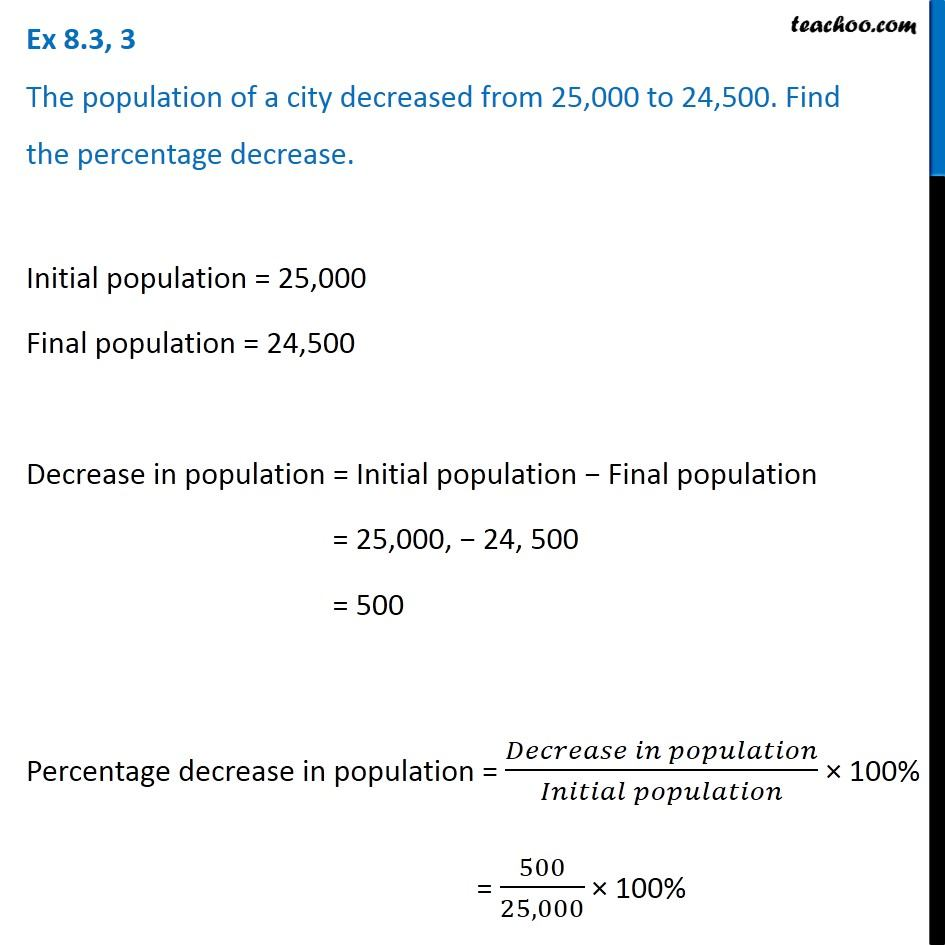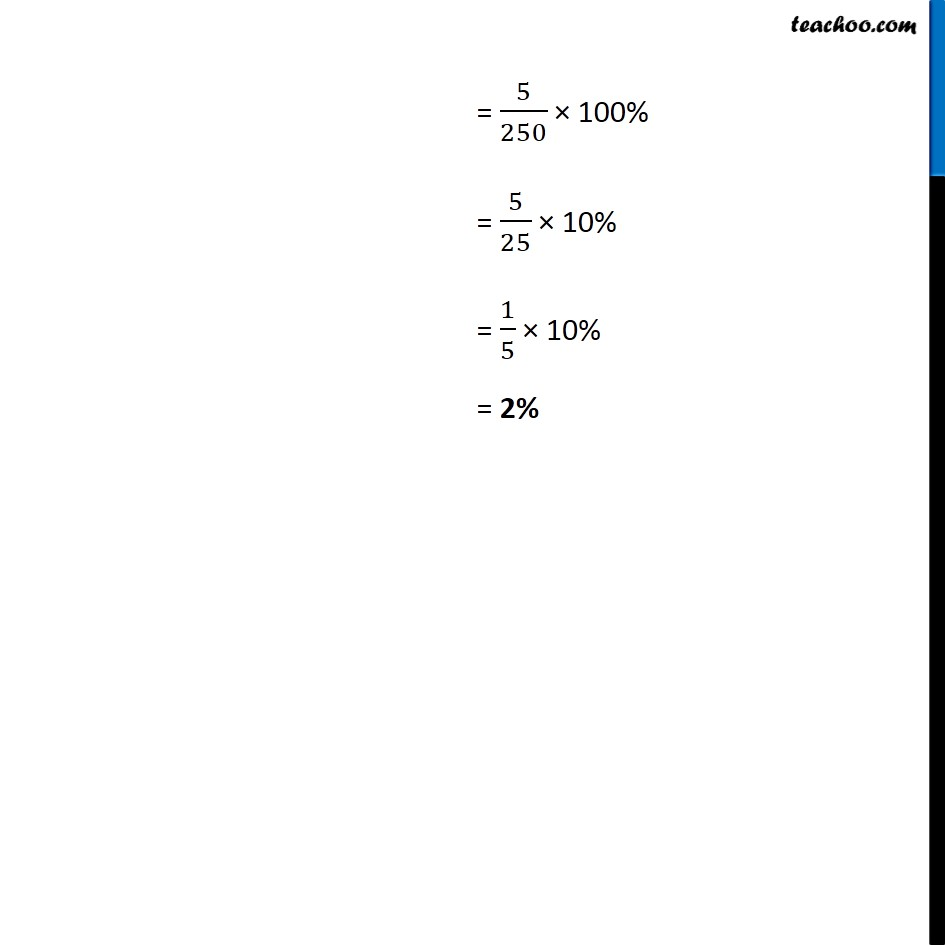Percentage increase or decrease

Chapter 8 Class 7 Comparing Quantities
Concept wiseIntroducing your new favourite teacher - Teachoo Black, at only ₹83 per month

### Transcript

Ex 8.3, 3 The population of a city decreased from 25,000 to 24,500. Find the percentage decrease. Initial population = 25,000 Final population = 24,500 Decrease in population = Initial population − Final population = 25,000, − 24, 500 = 500 Percentage decrease in population = (𝐷𝑒𝑐𝑟𝑒𝑎𝑠𝑒 𝑖𝑛 𝑝𝑜𝑝𝑢𝑙𝑎𝑡𝑖𝑜𝑛)/(𝐼𝑛𝑖𝑡𝑖𝑎𝑙 𝑝𝑜𝑝𝑢𝑙𝑎𝑡𝑖𝑜𝑛) × 100% = 500/25,000 × 100% = 5/250 × 100% = 5/25 × 10% = 1/5 × 10% = 2%